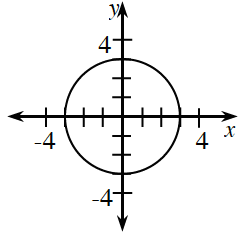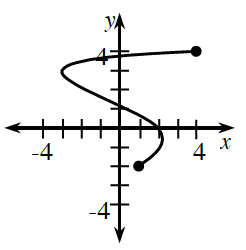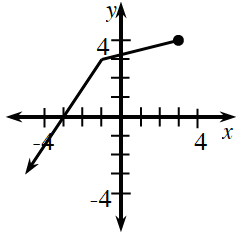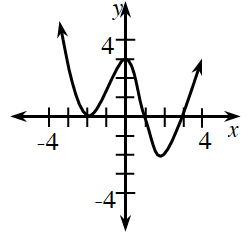### Home > INT3 > Chapter 4 > Lesson 4.1.2 > Problem4-24

4-24.

State the domain and range of each graph. Then state whether or not it is a function.

The domain is the set of all $x$-values of a relation or function.
The range is the set of all $y$-values of a relation or function.
For a relation to be a function, each $x$-value must correspond to only one $y$-value.

1.Domain: $−3\le x\le3$
Range: $−3\le y\le3$
Not a Function

1.Domain: $−3\le x\le4$
Find the range.
Is this a function?

1.Find the domain.
Range: $−∞\le y\le4$
Is this a function?

1.This is a function.
Find the domain and range.